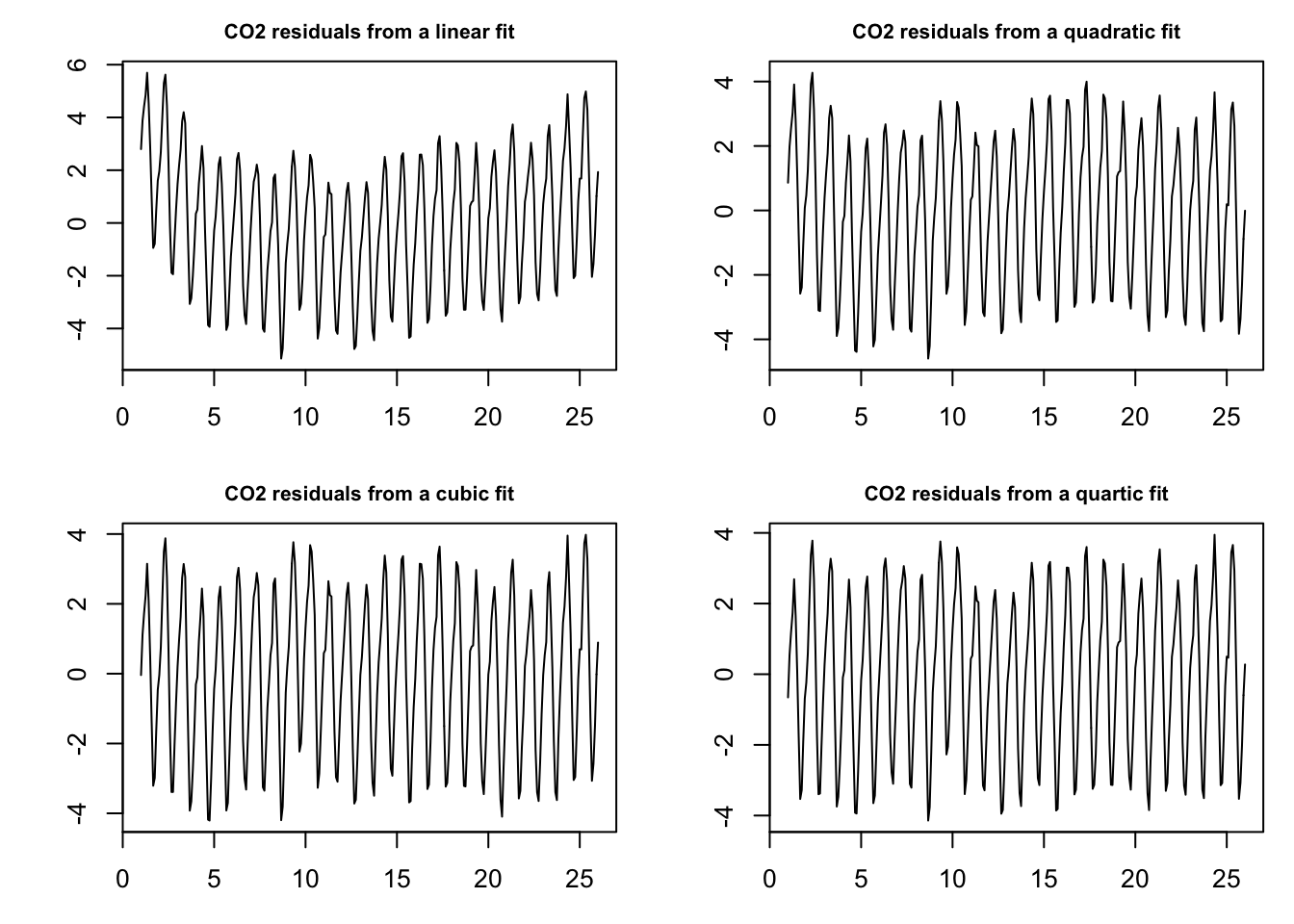#### Least squares estimation

Let us reload the working data set, the subset of the Keeling Cruve from 1990 to 2015, from the previous section.

``````library(xts)

We apply the least squares estimation and fit 4 different models, a linear \((p=1)\), a quadratic \((p=2)\), a cubic \((p=3)\) and a quartic \((p=4)\) model, by applying the `lm()` function.

``````t <- 1:length(co2.1990.2015)
fit1 <- lm(co2.1990.2015~t)
fit2 <- lm(co2.1990.2015~t+I(t^2))
fit3 <- lm(co2.1990.2015~t+I(t^2)+I(t^3))
fit4 <- lm(co2.1990.2015~t+I(t^2)+I(t^3)+I(t^4))``````

As the residuals from the fit yield a time series without the trend, we evaluate the goodness of the models by plotting the residuals.

``````list.fits <- list(fit1, fit2, fit3, fit4)
power <- c('linear', 'quadratic', 'cubic', 'quartic')

par(mfrow = c(2,2), mar = c(3,4,2,1))
for (i in 1:length(list.fits)){
r = list.fits[[i]]\$residuals
plot.ts(r,
ylab = '',
main = paste('CO2 residuals from a' , power[i], 'fit'),
cex.main = 0.8)
}``````The plots of residuals indicate that the linear fit (upper left) did not remove the entire trend in the data. There appears to be some nonlinearity to the trend. The residual plot from the quadratic fit (upper right) still showed some structure. The cubic model (lower left) and the quartic mode (lower right) seem to be a good fit to the data.

Following Ockham’s razor we pick the cubic model for further analysis. A summary of the cubic model is provided by calling `summary(fit3)`.

``summary(fit3)``
``````##
## Call:
## lm(formula = co2.1990.2015 ~ t + I(t^2) + I(t^3))
##
## Residuals:
##     Min      1Q  Median      3Q     Max
## -4.2066 -1.9454  0.2192  1.8019  3.9783
##
## Coefficients:
##               Estimate Std. Error t value Pr(>|t|)
## (Intercept)  3.538e+02  5.174e-01 683.717  < 2e-16 ***
## t            8.073e-02  1.481e-02   5.450 1.06e-07 ***
## I(t^2)       4.349e-04  1.139e-04   3.820 0.000163 ***
## I(t^3)      -6.739e-07  2.479e-07  -2.719 0.006943 **
## ---
## Signif. codes:  0 '***' 0.001 '**' 0.01 '*' 0.05 '.' 0.1 ' ' 1
##
## Residual standard error: 2.216 on 297 degrees of freedom
## Multiple R-squared:  0.9747, Adjusted R-squared:  0.9744
## F-statistic:  3811 on 3 and 297 DF,  p-value: < 2.2e-16``````

The p-values indicate that the estimated coefficients of the cubic fit are statistical significant.

#### Differencing

Alternatively, to least squares estimation we may apply differencing. For the purpose of demonstration we apply first order \((\Delta_{y_t})\) , second order \((\Delta_{y_t}^2)\), third order \((\Delta_{y_t}^3)\) and fourth order \((\Delta_{y_t}^4)\) differencing.

To difference a series, \(\Delta_{y_t}=y_t-y_{t-1}\), in R we apply the `diff()` function. Use `diff(y)` for \((\Delta_{y_t})\), `diff(diff(y))` for \((\Delta_{y_t}^2)\), `diff(diff(diff(y)))` for \((\Delta_{y_t}^3)\),and so on for higher order differencing.

``````diff1 <- diff(co2.1990.2015)
diff2 <- diff(diff(co2.1990.2015))
diff3 <- diff(diff(diff(co2.1990.2015)))
diff4 <- diff(diff(diff(diff(co2.1990.2015))))``````

Let us plot the results:

``````list.diffs <- list(diff1, diff2, diff3, diff4)
diff.level <-  c(1:4)
par(mfrow = c(2,2), mar = c(3,4,2,1))
for (i in 1:length(list.diffs)){
diff <- list.diffs[[i]]
plot.ts(diff,
ylab = '',
main = substitute(paste('Differencing: ', Delta^l,y[t]), list(l = diff.level[i])),
cex.main = 0.8)
}``````